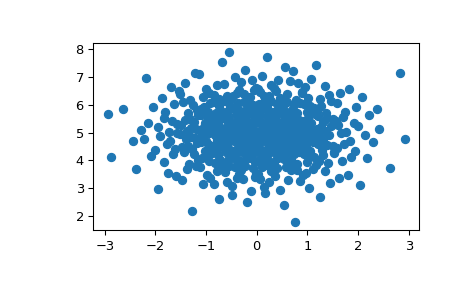# scipy.stats.qmc.MultivariateNormalQMC¶

class scipy.stats.qmc.MultivariateNormalQMC(mean, cov=None, *, cov_root=None, inv_transform=True, engine=None, seed=None)[source]

QMC sampling from a multivariate Normal $$N(\mu, \Sigma)$$.

Parameters
meanarray_like (d,)

The mean vector. Where d is the dimension.

covarray_like (d, d), optional

The covariance matrix. If omitted, use cov_root instead. If both cov and cov_root are omitted, use the identity matrix.

cov_rootarray_like (d, d’), optional

A root decomposition of the covariance matrix, where d' may be less than d if the covariance is not full rank. If omitted, use cov.

inv_transformbool, optional

If True, use inverse transform instead of Box-Muller. Default is True.

engineQMCEngine, optional

Quasi-Monte Carlo engine sampler. If None, Sobol is used.

seed{None, int, numpy.random.Generator}, optional

If seed is None the numpy.random.Generator singleton is used. If seed is an int, a new Generator instance is used, seeded with seed. If seed is already a Generator instance then that instance is used.

Examples

>>> import matplotlib.pyplot as plt
>>> from scipy.stats import qmc
>>> engine = qmc.MultivariateNormalQMC(mean=[0, 5], cov=[[1, 0], [0, 1]])
>>> sample = engine.random(512)
>>> _ = plt.scatter(sample[:, 0], sample[:, 1])
>>> plt.show()Methods

 Fast-forward the sequence by n positions. random([n]) Draw n QMC samples from the multivariate Normal. Reset the engine to base state.